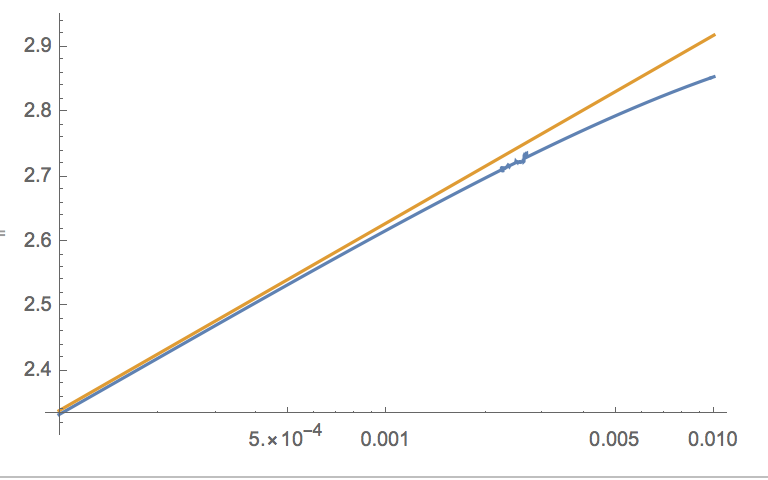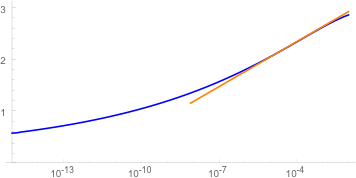# Getting asymptotic behaviour of an integral?

I am interested in the $\rho\sim0$ asymptotics of the following expression $$\int_{1.1}^{\infty}\frac{\sin(k\rho)}{k^{1.9}\rho}\frac{1}{\log\frac{1}{k}}\,dk$$ any ideas of how to tackle this?

• Have you tried to cut the tail of the integral (say, from 10000) and after that to replace $\sin (k\rho)=k\rho-\frac{k^3\rho^3} 6 +O((k\rho)^5)?$ Dec 29, 2016 at 18:07
• @user64494: This does not work. if we let $\rho\to 0$ under the integral, the resulting integral diverges! Dec 29, 2016 at 19:24
• @Michael Renardy : Could you elaborate and base your statement? Dec 29, 2016 at 19:57

Setting $t=\rho k$ the integral in question becomes $$\int_{1.1\rho}^\infty\frac{\sin t}{\rho^{0.1}t^{1.9}\log (t/\rho)}\;dt.$$ Fix $\epsilon>0$. Then for $\rho\rightarrow 0$ we have $$\int_{1.1\rho}^{\rho^{1/2}} \frac{\sin t}{t^{1.9}\log (t/\rho)}\;dt \ll \int_{1.1\rho}^{\rho^{1/2}} \frac{1}{t^{0.9}}\;dt \ll \rho^{1/20},$$ $$\int_{\rho^{1/2}}^\epsilon\frac{\sin t}{t^{1.9}\log (t/\rho)}\;dt \ll\frac{1}{\log\rho^{-1}}\int_{\rho^{1/2}}^\epsilon\frac{dt}{t^{0.9}}\ll\frac{\epsilon^{0.1}}{\log\rho^{-1}},$$ and $$\left|\int_{\epsilon^{-1}}^\infty\frac{\sin t}{t^{1.9}\log (t/\rho)}\;dt\right| \ll \int_{\epsilon^{-1}}^\infty\frac{1}{t^{1.9}\log \rho^{-1}}\;dt \ll\frac{\epsilon^{0.1}}{\log\rho^{-1}}.$$ Hence for $\rho<\epsilon^2$ the integral in question equals $$\rho^{-0.1}\int_\epsilon^{\epsilon^{-1}}\frac{\sin t}{t^{1.9}\log(t/\rho)}\;dt + \mathcal{O}\left(\frac{\epsilon^{0.1}\rho^{-0.1}}{\log\rho^{-1}}\right).$$ Now $$\int_\epsilon^{\epsilon^{-1}}\frac{\sin t}{t^{1.9}\log(t/\rho)}\;dt = \int_\epsilon^{\epsilon^{-1}}\frac{\sin t}{t^{1.9}(\log\rho^{-1} + \mathcal{O}(\log\epsilon^{-1}))}\;dt,$$ since in the range of integration we have $|\log t|\leq\log\epsilon^{-1}$. Putting $L=\log\rho^{-1}$ and $\alpha=\log\epsilon^{-1}$ we have for $\alpha<L/2$, i.e. $\rho<\epsilon^2$ $$\left|\frac{1}{L}-\frac{1}{L+\alpha}\right| = \frac{|\alpha|}{L(L+\alpha)} \ll \frac{|\alpha|}{L^2},$$ thus \begin{split} \int_\epsilon^{\epsilon^{-1}}\frac{\sin t}{t^{1.9}(L + \mathcal{O}(\alpha))}\;dt &= \int_\epsilon^{\epsilon^{-1}}\frac{\sin t}{t^{1.9}}\left(\frac{1}{L}+\mathcal{O}\left(\frac{\alpha}{L^2}\right)\right)\;dt\\ & = \frac{1}{L}\int_\epsilon^{\epsilon^{-1}}\frac{\sin t}{t^{1.9}}\;dt + \mathcal{O}\left(\frac{\alpha}{L^2}\int_\epsilon^{\epsilon^{-1}}\frac{|\sin t|}{t^{1.9}}\right). \end{split} In the error term we bound $|\sin t|$ by $\min(t, 1)$ and split the integral into the range $[\epsilon, 1]$ and $[1, \epsilon^{-1}]$. Doing so we find that the integral converges, hence the error term is $\mathcal{O}(\frac{\alpha}{L^2})$. In the main term we extend the integral to the range $(0,\infty)$. Doing so introduces an error $$\frac{1}{L}\int_0^\epsilon\frac{\sin t}{t^{1.9}}\;dt \leq \frac{1}{L}\int_0^\epsilon\frac{1}{t^{0.9}}\;dt\ll\frac{\epsilon^{0.1}}{L}$$ and another one of size $$\frac{1}{L}\int_{\epsilon^{-1}}^\infty\frac{\sin t}{t^{1.9}}\;dt \leq \frac{1}{L}\int_{\epsilon^{-1}}^\infty\frac{dt}{t^{1.9}} \ll \frac{\epsilon^{0.9}}{L}.$$ Together we obtain $$\int_\epsilon^{\epsilon^{-1}}\frac{\sin t}{t^{1.9}\log(t/\rho)}\;dt = \frac{1}{L}\int_0^\infty\frac{\sin t}{t^{1.9}}\;dt + \mathcal{O}\left(\frac{\epsilon^{0.1}}{L}+\frac{\alpha}{L^2}\right).$$ Together with the bounds obtained before we get that the original integral equals $$\frac{\rho^{-0.1}}{L}\int_0^\infty\frac{\sin t}{t^{1.9}}\;dt + \mathcal{O}\left(\frac{\rho^{-0.1}\epsilon^{0.1}}{L}+\frac{\rho^{-0.1}\alpha}{L^2}\right).$$ Putting $\epsilon=L^{10}$, i.e. $\alpha=10\log\log\rho^{-1}$, we get $$\frac{\rho^{-0.1}}{L}\int_0^\infty\frac{\sin t}{t^{1.9}}\;dt + \mathcal{O}\left(\frac{\rho^{-0.1}\log\log\rho^{-1}}{(\log\rho^{-1})^2}\right).$$ The integral in the main term is positive, thus the main term is larger than the error term by a factor $\frac{\log\log\rho^{-1}}{\log\rho^{-1}}$, and we got an asymptotic formula.

The main source of error is the approximation of the term $\log\rho^{-1}+t$ by $\log\rho^{-1}$. If you need better asymptotics, you would have to use the series expansion of $\frac{1}{1+x}$. In this way you would get an asymptotic series in $\frac{1}{L}$, but the computations would become rather long.

• How do you justify $\int_{\epsilon}^{\epsilon^{-1}}\frac{\sin t}{t^{1.9}\log\frac{t}{\rho}}dt=\int_{\epsilon}^{\epsilon^{-1}}\frac{\sin t}{t^{1.9}[\log\rho^{-1}+\mathcal{O}(\log\epsilon^{-1})]}dt$? Jan 7, 2017 at 15:15
• We have $\epsilon\leq t\leq\epsilon^{-1}$, thus $\log(t/\rho)=\log\rho^{-1}+\log t=\log\rho^{-1}+\mathcal{O}(\log\epsilon^{-1})$. Jan 7, 2017 at 16:49
• Good thanks. Now, how do you justify $\int_{\epsilon}^{\epsilon^{-1}}\frac{\sin t}{t^{1.9}[\log\rho^{-1}+\mathcal{O}(\log\epsilon^{-1})]}dt=\frac{1}{\log\rho^{-1}}\int_{\epsilon}^{\epsilon^{-1}}\frac{\sin t}{t^{1.9}}dt+\mathcal{O}\bigg(\frac{\log\epsilon^{-1}}{\log^2\rho^{-1}}\int_{\epsilon}^{\epsilon^{-1}}\frac{|\sin t|}{t^{1.9}}dt\bigg)$? Jan 7, 2017 at 17:07
• I don't wanna annoy you but everything that goes beyond where you say "Now ..." puzzles me. My knowledge of asymptotic analysis is very elementary and I would appreciate more indications Jan 7, 2017 at 17:16
• I expanded the computations, stretching the performance of MathJax to the limits of my computer. Jan 7, 2017 at 18:59

the small-$$\rho$$ asymptotics of $$I(\rho)=-\int_{1.1}^{\infty}\frac{\sin(k\rho)}{\rho k^{1.9}\ln k}\,dk$$

is governed by the large-$$k$$ behavior of the integrand, which gives $$I(\rho)\propto \rho^{1.9-2}$$ up to a logarithmic factor, and a numerical evaluation supports the asymptotic

$$I_{\rm asymp}(\rho)=-\rho^{-0.1}(3.5+0.126\ln\rho)$$Log-linear plot of $$-\rho^{0.1}I(\rho)$$ (blue line) and $$-\rho^{0.1}I_{\rm asymp}(\rho)$$ (orange line)

UPDATE, following Bazin's recent answer:

I have some convergence issues with a numerical evaluation at much smaller $$\rho$$, but if I can trust the Mathematica output the curve does seem to level off towards an asymptote at zero, as derived by Bazin, possibly as $$I(\rho)=-\text{constant}\times(\rho^{0.1}\log\rho)^{-1}$$.• Can you present these numerics (possibly, through Dropbox)? Dec 29, 2016 at 18:43
• @user64494 --- added a plot in support of the asymptotics Dec 29, 2016 at 21:41
• @CarloBeenakker any analytic argument leading to this behavior? Dec 30, 2016 at 11:40
• That's nice. A change of variable $x = \rho k$ converts the integral to a tractable looking integrable that I think gives the same result.
– user83457
Dec 30, 2016 at 13:29

Let us define $\epsilon_0=0.1$, so that $I(x)=- x^{-1}\Im\int_{\mathbb R}\frac{H(t-1-\epsilon_0)e^{itx}}{t^{2-\epsilon_0}\ln t} dt.$ We get for $x>0$, $$I(x)=-x^{-1}\int_{x(1+\epsilon_0)}^{+\infty} \tau^{-2+\epsilon_0}x^{2-\epsilon_0}\frac{\sin{\tau}}{\ln \tau-\ln x}x^{-1}d\tau=- x^{-\epsilon_0}\int_{x(1+\epsilon_0)}^{+\infty} \frac{\sin \tau}{\tau^{2-\epsilon_0}}\frac{d\tau}{\ln \tau-\ln x}.$$ We note that $\ln \tau-\ln x\ge \ln (1+\epsilon_0)=\epsilon_1>0.$ On the other hand the function $$\tau\mapsto \frac{\sin \tau}{\tau^{2-\epsilon_0}}\quad \text{belongs to L^1(\mathbb R_+)},$$ so that the Lebesgue Dominated Convergence Theorem gives $\lim_{x\rightarrow 0_+}I(x)x^{\epsilon_0}=0.$ Defining $$I_1(x)=-x^{-\epsilon_0}\int_{1}^{+\infty} \frac{\sin \tau}{\tau^{2-\epsilon_0}}\frac{d\tau}{\ln \tau-\ln x},$$ and noticing that $\ln \tau-\ln x\ge \ln(1/x)$ for $\tau \ge 1$, the same argument as above gives $$I_1(x)\sim_{x\rightarrow 0_+} -\frac{1}{x^{\epsilon_0}\ln (1/x)} \int_{1}^{+\infty} \frac{\sin \tau}{\tau^{2-\epsilon_0}}d\tau.$$ We set $I_0(x)= - x^{-\epsilon_0}\int_{x(1+\epsilon_0)}^{1} \frac{\sin \tau}{\tau^{2-\epsilon_0}}\frac{d\tau}{\ln \tau-\ln x}.$ We know that $\lim_{x\rightarrow 0_+} I_0(x) x^{\epsilon_0}=0$ and it remains to find an equivalent for $I_0(x)$ for $x\rightarrow 0_+$.

• I extended the numerics, in support of your conclusion, thanks. Jan 6, 2017 at 19:01
• @CarloBeenakker I completed my answer, but left a question for a remaining integral. Jan 7, 2017 at 10:49

Split the integral at $k \approx 1/\rho$. For $k \leq 1/\rho$ approximate $\sin(k \rho) \approx k \rho$ by it's Taylor expansion (just the first term suffices) and compute what you get, this is your main term . In the region $k \geq 1 / \rho$ make a linear change of variable $k \rho \rightarrow k$ and notice that this part of the integral vanishes as $\rho$ goes to zero, this is your error term .

• I don't see how the second part vanishes when $\rho\to 0$, actually I think it is not the case Dec 30, 2016 at 9:17
• yes you're right i was too careless when making my change of variable, the other part contributes about an equal amount. Dec 30, 2016 at 23:20